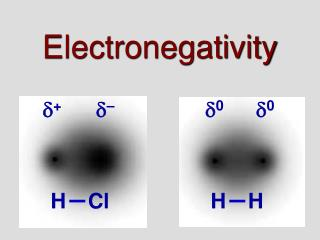DownloadDownload PresentationElectronegativity

# Electronegativity

Télécharger la présentation## Electronegativity

- - - - - - - - - - - - - - - - - - - - - - - - - - - E N D - - - - - - - - - - - - - - - - - - - - - - - - - - -
##### Presentation Transcript

1. Electronegativity + – 0 0 H Cl H H

2. H H H H H H Na Na Na Na Cl Cl Cl Cl O O O The basic units: ionic vs. covalent • Ionic compounds form repeating units. • Covalent compounds form distinct molecules. • Consider adding to NaCl(s) vs. H2O(s): • NaCl: atoms of Cl and Na can add individually forming a compound with million of atoms. • H2O: O and H cannot add individually, instead molecules of H2O form the basic unit.

3. Intramolecular forces occur between atoms Intermolecular forces occur between molecules Holding it together Q: Consider a glass of water. Why do molecules of water stay together? A: there must be attractive forces. Intramolecular forces are much stronger • We do not consider intermolecular forces in ionic bonding because there are no molecules. • We will see that the type of intramolecular bond determines the type of intermolecular force.

4. + + 0 – 0 – H2 HCl LiCl H H Cl H [Cl]– [Li]+ I’m not stealing, I’m sharing unequally • We described ionic bonds as stealing electrons • In fact, all bonds share – equally or unequally. • Note how bonding electrons spend their time: covalent (non-polar) polarcovalent ionic • Point: the bonding electrons are shared in each compound, but are not always shared equally. • The greek symbol  indicates “partial charge”.

5. Electronegativity • Recall that electronegativity is “a number that describes the relative ability of an atom, when bonded, to attract electrons”. • The periodic table has electronegativity values. • We can determine the nature of a bond based on EN (electronegativity difference). • EN = higher EN – lower EN NBr3: EN = 3.0 – 2.8 = 0.2 (for all 3 bonds). • Basically: a EN below 0.5 = covalent, 0.5 - 1.7 = polar covalent, above 1.7 = ionic • Determine the EN and bond type for these: HCl, CrO, Br2, H2O, CH4, KCl

6. Electronegativity Answers HCl: 3.0 – 2.1 = 0.9 polar covalent CrO: 3.5 – 1.6 = 1.9 ionic Br2: 2.8 – 2.8 = 0 covalent H2O: 3.5 – 2.1 = 1.4 polar covalent CH4: 2.5 – 2.1 = 0.4 covalent KCl: 3.0 – 0.8 = 2.2 ionic

7. + + + – + – – – + – + – Electronegativity & physical properties CaCl2 would have a lower melting/boiling point: CaCl2 = 3.0 – 1.0 = 2.0 CaF2 = 4.0 – 1.0 = 3.0 Note: other factors such as atomic size within molecules also affects melting and boiling points. EN is an important factor but not the only factor. It is most useful when comparing atoms and molecules of similar size. • Electronegativity can help to explain properties of compounds like those in the lab. • Lets look at HCl: partial charges keep molecules together. LiBr would have a lower melting/boiling point: KCl = 3.0 – 0.8 = 2.2 LiBr = 2.8 – 1.0 = 1.8 • The situation is similar in NaCl, but the attraction is even greater (EN = 2.1 vs. 0.9 for HCl). H2S would have a lower melting/boiling point: H2O= 3.5 – 2.1 = 1.4 H2S = 2.5 – 2.1 = 0.4 • Whichwouldhaveahighermelting/boilingpoint? • NaCl because of its greater EN. • For each, pick the one with the lower boiling point a) CaCl2, CaF2 b) KCl, LiBr c) H2O, H2S

8. CaCl2 would have a lower melting/boiling point: CaCl2 = 3.0 – 1.0 = 2.0 CaF2 = 4.0 – 1.0 = 3.0 Note: other factors such as atomic size within molecules also affects melting and boiling points. EN is an important factor but not the only factor. It is most useful when comparing atoms and molecules of similar size. LiBr would have a lower melting/boiling point: KCl = 3.0 – 0.8 = 2.2 LiBr = 2.8 – 1.0 = 1.8 H2S would have a lower melting/boiling point: H2O= 3.5 – 2.1 = 1.4 H2S = 2.5 – 2.1 = 0.4

9. – – – – – – – – – + + + + + + + + + + + + + + + + + + Why oil and water don’t mix • Lets take a look at why oil and water don’t mix (oil is non-polar, water is polar) The partial charges on water attract, pushing the oil (with no partial charge) out of the way.